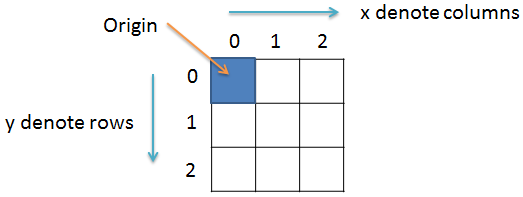# How to convert a color image into negative

Image Processing Project

In this project we will learn to convert a color image into its negative using Java programming language.

### Prerequisite

It is assumed that you have completed the projects titled How to read and write image file in Java and How to get and set pixel value in Java before starting this project.

### Color image to negative

Converting a color image into negative is very simple. All we have to do is repeat 3 simple steps for each pixels of the image.

1. Get the RGB value of the pixel.
2. Calculate the new RGB value as shown below.

R = 255 – R
G = 255 – G
B = 255 – B

3. Save the new RGB value in the pixel.

Example:

Consider a color pixel with the following values

A = 255

R = 100

G = 150

B = 200

Where A, R, G and B represents the Alpha, Red, Green and Blue value of the pixel.

Remember! ARGB will have an integer value in the range 0 to 255.

So, to convert the color pixel into negative we will subtract the value of R, G and B from 255.

R = 255 - 100 = 155

G = 255 - 150 = 105

B = 255 - 200 = 55

So, the new ARGB value will be:

A = 255

R = 155

G = 105

B = 55

Note! We don't have to change the alpha value because it only controls the transparency of the pixel.

### Import the required classes

Create a new file and save it by the name Negative.java. Open the file and import the following:

``import java.io.File;``import java.io.IOException;``import java.awt.image.BufferedImage;``import javax.imageio.ImageIO;``

### Create the class

Next we will create the class by the name Negative and inside the class we will have the main() method. For this we will write:

``public class Negative{``  public static void main(String args[])throws IOException{``  // some code goes here...  }//main() ends here}//class ends here``

### Variables

Inside the main() method we create a BufferedImage variable img and a File variable f and set both of them to null. The img variable will hold the image file while the f variable will hold the image file path.

``BufferedImage img = null;``File f = null;``

For this project we will read the color image Taj.jpg and it is inside the Image folder which is inside D: drive on a Windows PC. So, we will first create an object of File class and pass as parameter the image file path. And then we will read the image file by calling the read() method of ImageIO class. So, our code will look something like the following:

``//read image``try{``  f = new File("D:\\Image\\Taj.jpg");``  img = ImageIO.read(f);``}catch(IOException e){``  System.out.println(e);``}``

### Get image dimensions

Now that we have the image stored in the img variable, it's time for us to find the dimensions of the image. For this we will create two integer variable width and height and use the getWidth() andgetHeight() method to get the width and height of the image respectively. So, we will write the following lines.

``//get image width and height``int width = img.getWidth();``int height = img.getHeight();``

### Negative code

We know that an image is made up of pixels which we can represent in 2D co-ordinate. So, we will create two variables x and y and use two for loops to traverse each pixels.For this we will write:

``for(int y = 0; y < height; y++){``  for(int x = 0; x < width; x++){  // some code goes here...  }}``

### Get pixel value and extract ARGB value

Inside the for loop we will first get the value of the pixel at co-ordinate (x,y) using thegetRGB(x,y) method. Then we will extract the ARGB value from the pixel value. For this purpose we will be using 5 integer values p, a, r, g and b.

``int p = img.getRGB(x,y);``int a = (p>>24)&0xff;``int r = (p>>16)&0xff;``int g = (p>>8)&0xff;``int b = p&0xff;``

### Set new pixel value

To convert the color image into its negative we have to subtract the R, G and B value from 255. So we will write the following line of code.

``r = 255 - r;``g = 255 - g;``b = 255 - b;``

And, now we will set the new pixel value. For this we will write:

``p = (a<<24) | (r<<16) | (g<<8) | b;``img.setRGB(x, y, p);``

### Write image

After the for loop ends we will write the image file. For this we will write the following code:

``try{``  f = new File("D:\\Image\\Output.jpg");``  ImageIO.write(img, "jpg", f);``}catch(IOException e){``  System.out.println(e);``}``

### Final code

``/**`` * File: Negative.java`` * `` * Description:`` * Convert color image to negative.`` * `` * @author Yusuf Shakeel`` * Date: 27-01-2014 mon`` *`` * www.github.com/yusufshakeel/Java-Image-Processing-Project`` */``import java.io.File;``import java.io.IOException;``import java.awt.image.BufferedImage;``import javax.imageio.ImageIO;``public class Negative{``  public static void main(String args[])throws IOException{``    BufferedImage img = null;``    File f = null;``    //read image``    try{``      f = new File("D:\\Image\\Taj.jpg");``      img = ImageIO.read(f);``    }catch(IOException e){``      System.out.println(e);``    }``    //get image width and height``    int width = img.getWidth();``    int height = img.getHeight();``    //convert to negative``    for(int y = 0; y < height; y++){``      for(int x = 0; x < width; x++){``        int p = img.getRGB(x,y);``        int a = (p>>24)&0xff;``        int r = (p>>16)&0xff;``        int g = (p>>8)&0xff;``        int b = p&0xff;``        //subtract RGB from 255``        r = 255 - r;``        g = 255 - g;``        b = 255 - b;``        //set new RGB value``        p = (a<<24) | (r<<16) | (g<<8) | b;``        img.setRGB(x, y, p);``      }``    }``    //write image``    try{``      f = new File("D:\\Image\\Output.jpg");``      ImageIO.write(img, "jpg", f);``    }catch(IOException e){``      System.out.println(e);``    }``  }//main() ends here``}//class ends here``